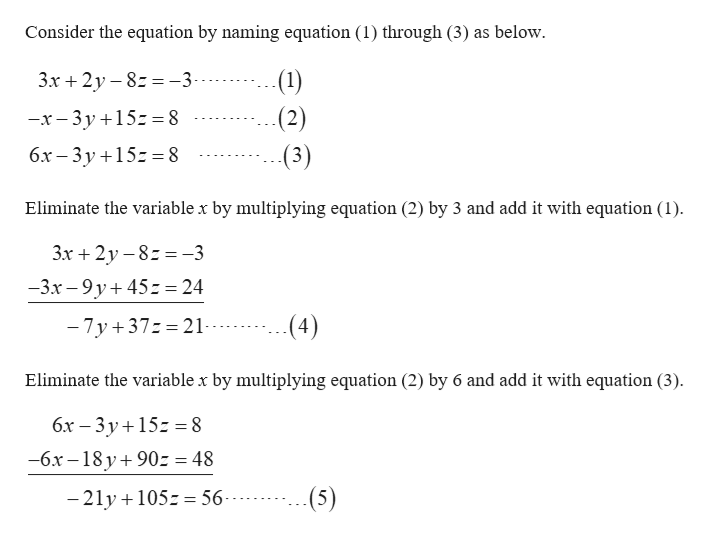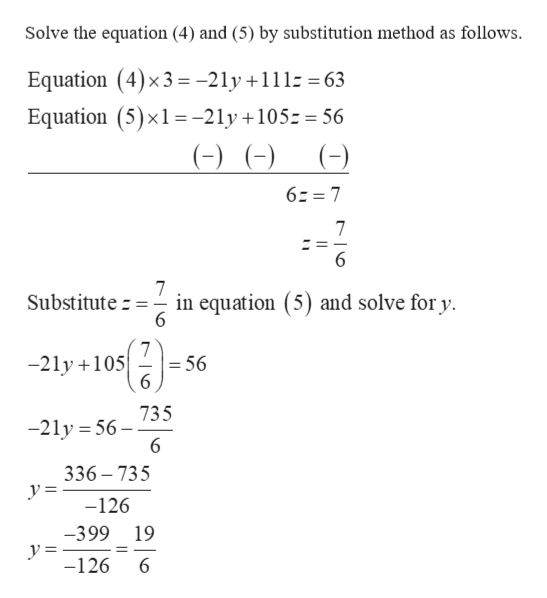# 3x+2y-8z=-3-x-3y+15z=86x-3y+15z=8Solve equations either by elimination or substitution

Question
1 views

3x+2y-8z=-3

-x-3y+15z=8

6x-3y+15z=8

Solve equations either by elimination or substitution

check_circle

Step 1help_outlineImage TranscriptioncloseConsider the equation by naming equation (1) through (3) as below (1) 3.x2y8-3 (2) -x-3y +15-8 бх — 3у+15-%38 (3) Eliminate the variable x by multiplying equation (2) by 3 and add it with equation (1) Зх + 2у -8-%3-3 -3x-9y45 = 24 - 7y 37 21- (4) Eliminate the variable x by multiplying equation (2) by 6 and add it with equation (3) бх — 3у+15: %3D8 -6x -18 y90 = 48 (5) - 21y 105 56-- fullscreen
Step 2help_outlineImage TranscriptioncloseSolve the equation (4) and (5) by substitution method as follows Equation (4)x3= -2ly +111 63 Equation (5) x1 =-2ly +105 - 56 ( ( (-) 62 7 7 7 Substitute in equation (5) and solve for y 6 7 -21y 105 = 56 6 735 -21y 56 6 336 735 y = -126 -399 19 y = -126 6 fullscreen

### Want to see the full answer?

See Solution

#### Want to see this answer and more?

Solutions are written by subject experts who are available 24/7. Questions are typically answered within 1 hour.*

See Solution
*Response times may vary by subject and question.
Tagged in

### Other